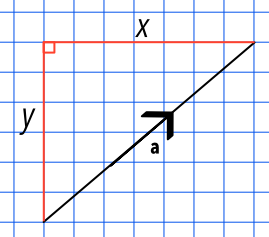Magnitude of a Vector

## Magnitude of a Vector

A column vector gives both magnitude and direction. The magnitude, or size, of a vector is written as |a| (called modular a, when the sign is ignored). When considering just the size (magnitude) of the vector, the direction is irrelevant.

Because a column vector uses rectangular components (ie at right angles) the magnitude of the vector can be obtained using Pythagoras: for a vector ((x),(y)), this is sqrt(x^2 + y^2).## Example 1

What is the magnitude of the resultant vector 3bb(g) + 2bb(h), when bbg = ((2),(5)) and bb(h) = ((1),(-1))?

The resultant vector is ((3 xx 2 + 2 xx 1),(3 xx 5 + 2 xx -1)) = ((8),(13))

Using Pythagoras: a^2 = b2 + c^2

= sqrt(8^2 + 13^2)

= 15.264

=15.26 (2dp)

## Example 2

Vector c is defined as ((7),(11)).

What is the magnitude of c?

Use Pythagoras to determine the magnitude:

magnitude = sqrt(7^2 + 11^2)

= 13.038